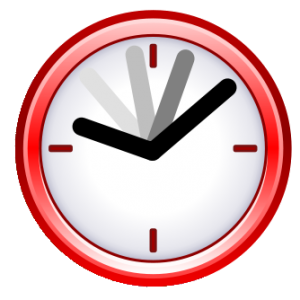# Modular Arithmetic & Fermat’s Little Theorem

by | Jan 12, 2021 | Math Learning

Modular arithmetic is a way of counting in which the numbers wrap around after reaching a certain value. The clock is often used as an analogy. While time always progresses forward, the 12-hour clock “resets” to 1 after passing 12 (13 o’clock is equivalent to 1 o’clock). If we replace 12 with 0, we have the set known as ℤ12 which is made up of {0, 1, 2, 3, 4, 5, 6, 7, 8, 9, 10, 11}. If we consider midnight as t0=0, then 5 hours later is t5=5. 17 hours later will also be t17=5. 84 hours later will be t84 = 0. 85 hours later will be t85 = 1. There is a pattern here. The value a in the set ℤ12 corresponding to any other integer b is the remainder when b is divided by 12. We can write this mathematically as b ≡ a (mod 12), and we say “b is congruent to a modulo 12”A clock represents modular arithmetic in ℤ12

More generally, we can write the set of integers for any modulus n as ℤn = {0, 1, 2, 3, … n-1} . Each element a in ℤn represents not only a itself, but all of the b’s for which b ≡ a (mod n). These elements are called congruence classes, and the set ℤn is a set made up of these classes. Sometimes, it’s more convenient to write the relationship as b-a ≡ 0 (mod n), i.e. each b is assigned to a congruence class depending on how much needs to be subtracted to be divisible by n. Addition and multiplication can be done using modular arithmetic if you are using the same modulus. If b ≡ a (mod n) and d ≡ c (mod n), then

1. (b+d) ≡ (a+c) (mod n)
2. (b*d) ≡ (a*c) (mod n)

Example 1. What is 84,872 (mod 5)? A simple way to approach this problem is to find the closest multiple of 5 to 84,872. That is 84,870. Therefore, we can write 84,872 (mod 5) ≡ 84,870 (mod 5) + 2 (mod 5) ≡ 0 (mod 5) + 2 (mod 5) ≡ 2 (mod 5). Example 2. In modulo 10, what is 19,374 · 3,172? One way to attempt this problem is to multiply out these numbers and then find the remainder when dividing by 10. However, since we want our answer in modulo 10, we can instead multiply just the representatives from their congruence classes. The remainder when 19,374 is divided by 10 is 4, so 19,374 ≡ 4 (mod 10) and 3,172 ≡ 2 (mod 10), so (19,374 · 3,172) ≡ (2·4) (mod 10) ≡ 8 (mod 10). Example 3. In modulo 4, what is 180,700,247 + 64,987,422? Again we first find the congruence class for each number modulo 4. We can simplify each by breaking it into the sum of smaller multiples of 4. One possible way is to write 180,700,247 ≡ 180,700,200 (mod 4) + 44 (mod 4) + 3 (mod 4) ≡ 0 (mod 4) + 0 (mod 4) + 3 (mod 4) ≡ 3 (mod 4) Similarly, 64,987,422 ≡ 2 (mod 4). Therefore, (180,700,247+ 64,987,422) ≡ (3 + 2) (mod 4) ≡ 5 (mod 4). However, 5 is not in the set ℤ4 = {0, 1, 2, 3}, so we must reduce 5 (mod 4) to something in the set. We can simplify by noticing that 5 (mod 4) ≡ 4 (mod 4) + 1 (mod 4) ≡ 0 (mod 4) + 1 (mod 4) ≡ 1 (mod 4).

## Fermat’s Little Theorem

One important application for modular arithmetic is Fermat’s Little Theorem which states that if p is a prime number and a is not divisible by p, then ap-1 ≡ 1 (mod p). This theorem is useful because allows you to find a remainder when dividing a really big number by a prime number. Example 4. What is the remainder when 2784 is divided by 11? 2784 is too big to put in most calculators and too time-consuming to multiply by hand. We can apply Fermat’s Little Theorem to make it easier to solve. From the theorem we know that 210 ≡ 1 (mod 11). And we also know that we can multiply congruence classes with the same modulus. Therefore, one strategy we can apply is to write 2784 as the product of as many 210’s as possible. We can simplify as follows: 2784= (210)78·(24) ≡ 178 · 24 (mod 11) ≡ 24 (mod 11) ≡16 (mod 11) ≡ 5 (mod 11). The remainder is 5.Featured Bundles